• 先上一张评分卡 一、评分卡逻辑 ...评分卡中不直接用客户违约率p，而是用违约概率与正常概率的比值，称为Odds，即 Odds=p1−pOdds=\frac{p}{1-p}Odds=1−pp​， p=Odds1+Oddsp=\frac{Odds}{1+Od...
先上一张评分卡一、评分卡逻辑
信贷业务评估的是客户的客户违约率（Percent of Default）即PD，是[0,1]的概率，比如2%即100个客户中有2个违约，简称为p。
评分卡中不直接用客户违约率p，而是用违约概率与正常概率的比值，称为Odds，即

O

d

d

s

=

p

1

−

p

Odds=\frac{p}{1-p}

，

p

=

O

d

d

s

1

+

O

d

d

s

p=\frac{Odds}{1+Odds}

评分卡的背后逻辑是Odds的变动与评分变动的映射（把Odds映射为评分），分值是根据Odds的前提条件算出来的，不是人工取的。以单个客户在整张评分卡的得分的变动（比如评分从50分上升到70分）来反映Odds的变动（比如Odds从5%下降至1.25%），以及背后相对应的客户违约率PD的变动（比如从4.8%下降到1.2%）。违约率PD不直观、业务看起来不方便、不便计算，而评分就很直观、便于计算。如图所示。因此评分卡的生成过程，就是Odds变动映射成评分变动的过程。
二、评分映射公式
Odds映射为评分的公式为：

S

c

o

r

e

=

A

−

B

l

o

g

(

p

1

−

p

)

Score=A-Blog(\frac{p}{1-p})

<1> 预设条件
要算出系数A、B的话，需要从业务角度先预设两个前提条件：
在某个特定的比率

θ

0

\theta_0

设定特定的预期分值

P

0

P_0

指定比率翻番时分数的变动值（PDO）

解释：
比如根据业务经验，消费金融信贷的客户违约率4.8%算正常（

θ

0

=

O

d

d

s

=

5

%

)

）

。

预

设

评

分

卡

的

分

值

为

0

−

100

分

，

那

取

预

期

分

值

\theta_0=Odds=5\%)）。预设评分卡的分值为0-100分，那取预期分值

P_0)为50分，并指定当Odds按双倍上下浮动时（比如2.5%或10%），分值则对应上下变动10分（比如60分或40分）。这里

θ

0

\theta_0

=5%是根据业务经验来的，没有数学依据；0-100分是根据做评分卡的需要来的，没有数学依据。要是想做成600-1000分的评分卡也可以，修改对应的

P

0

P_0

和PDO就行；

P

0

P_0

=50分是根据0-100分来的，也可以取45分或73分，不重要。重要的是随着Odds翻番变动时，分数也随之变动的联动变化体系（你翻番我就变PDO=10分）

<2> 求解A、B
设定好

θ

0

\theta_0

、

P

0

P_0

、PDO后，联动变化为：

O

d

d

s

(

θ

0

)

Odds(\theta_0)

对应的分值为

P

0

P_0

，且翻番的

O

d

d

s

(

2

θ

0

)

Odds(2\theta_0)

对应的分值为

P

0

P_0

+PDO。则有以下两式：

P

0

=

A

−

B

l

o

g

(

θ

0

)

P_0=A-Blog(\theta_0)

P

0

+

P

D

O

=

A

−

B

l

o

g

(

2

θ

0

)

P_0+PDO=A-Blog(2\theta_0)

解出A、B为：

B

=

P

D

O

l

o

g

(

2

)

B=\frac{PDO}{log(2)}

A

=

P

0

+

B

l

o

g

(

θ

0

)

A=P_0+Blog(\theta_0)

按上面的解释举个例子：
设

θ

0

\theta_0

、

P

0

P_0

、PDO为5%、50分、10分，则

B

=

10

l

n

(

2

)

=

14.43

B=\frac{10}{ln(2)}=14.43

A

=

50

+

14.43

∗

l

n

(

0.05

)

=

6.78

A=50+14.43*ln(0.05)=6.78

则

S

c

o

r

e

=

6.78

−

14.43

l

o

g

(

p

1

−

p

)

Score=6.78-14.43log(\frac{p}{1-p})

<3> 完整的对应关系表
按照公式，可以把所有

O

d

d

s

(

p

1

−

p

)

Odds(\frac{p}{1-p})

和客户评分、客户违约概率（PD）的对应关系算出来该关系对应表应该算信用评分卡的核心思想了，评分是外层表现，客户违约率是内层核心，Odds是中间层转换计算

三、Odds映射X变量
那问题来了，现在能算Score了，但输入是Odds。但数据的输入是特征变量

[

x

1

,

x

2

,

x

3

,

⋯
&ThinSpace;

,

x

n

]

[x_1,x_2,x_3,\cdots,x_n]

，这里怎么对应呢？这就要说到逻辑回归本身了，先放结论：

l

o

g

(

p

1

−

p

)

=

β

0

+

β

1

x

1

+

β

2

x

2

+

⋯

+

β

n

x

n

log(\frac{p}{1-p})=\beta_0+\beta_1x_1+\beta_2x_2+\cdots+\beta_nx_n

怎么来的，以下详细讲。
<1> Sigmoid
逻辑回归来源于线性回归（二维空间中就是一条直线拟合所有样本点），虽然线性回归是回归算法，逻辑回归是分类算法，但从算法表达式上，逻辑回归就是在线性回归算法外面套了一层壳。
线性回归：

f

(

x

)

=

β

0

+

β

1

x

1

+

β

2

x

2

+

⋯

+

β

n

x

n

f(x)=\beta_0+\beta_1x_1+\beta_2x_2+\cdots+\beta_nx_n逻辑回归：

f

(

x

)

=

1

1

+

e

−

(

β

0

+

β

1

x

1

+

β

2

x

2

+

⋯

+

β

n

x

n

)

f(x)=\frac{1}{1+e^{-(\beta_0+\beta_1x_1+\beta_2x_2+\cdots+\beta_nx_n)}}可以看到，从表达式上看，逻辑回归只是在线性回归的表达式外面套了一层

f

(

x

)

=

1

1

+

e

−

x

f(x)=\frac{1}{1+e^{-x}}

的壳。为什么要套这层壳，因为线性回归的值域为实数集R，但逻辑回归是二分类算法，需要输出的是类别1和类别2的概率，而概率是个[0, 1]之间的数。因此需要将线性回归的输出实数变成[0, 1]之间的概率，而能满足输入是实数而输出是[0, 1]的，就是Sigmoid函数，它的图形是个类S（见上面逻辑回归图）的限定在[0, 1]之间的函数。因此将Sigmoid函数套在线性回归外面，构成逻辑回归，拥有处理非线性的能力，可以做分类。
<2> 变换公式形式
那么在信贷评分卡上，

f

(

x

)

f(x)

即为要预测的客户违约率（PD）

p

p

，另将

β

0

+

β

1

x

1

+

β

2

x

2

+

⋯

+

β

n

x

n

\beta_0+\beta_1x_1+\beta_2x_2+\cdots+\beta_nx_n

简写为向量形式

β

T

x

\beta^Tx

，即：

p

=

1

1

+

e

−

β

T

x

p=\frac{1}{1+e^{-\beta^Tx}}

经过变换，可得

l

n

(

p

1

−

p

)

=

β

T

x

ln(\frac{p}{1-p})=\beta^Tx

四、X变量细分到分组
好，回到主线，

l

o

g

(

p

1

−

p

)

=

β

0

+

β

1

x

1

+

β

2

x

2

+

⋯

+

β

n

x

n

log(\frac{p}{1-p})=\beta_0+\beta_1x_1+\beta_2x_2+\cdots+\beta_nx_n

，将score公式中的输入p变成输入特征变量X。到这里按理就可以结束了，有X就可以产出客户的Score，即：

S

c

o

r

e

=

A

−

B

(

β

0

+

β

1

x

1

+

β

2

x

2

+

⋯

+

β

n

x

n

)

Score=A-B(\beta_0+\beta_1x_1+\beta_2x_2+\cdots+\beta_nx_n)

但我们要做的是分组评分卡，X是要对应到每个分组，得到各变量分组的评分然后相加得到客户总评分的，那就还需要将X打散到各分类（用离散型数据入逻辑回归模型）。因此这里的输入X就不能是原始变量，而是原始变量分箱并算WOE后的woe值（类似离散变量中各类别的数值化），即：

S

c

o

r

e

=

A

−

B

[

β

0

+

β

1

(

δ

11

w

11

+

δ

12

w

12

+

δ

13

w

13

)

+

β

2

(

δ

21

w

21

+

δ

22

w

22

+

δ

23

w

23

+

δ

24

w

24

)

+

⋯

+

β

n

(

δ

n

1

w

n

1

+

δ

n

2

w

n

2

)

]

Score=A-B[\beta_0+\beta_1(\delta_{11}w_{11}+\delta_{12}w_{12}+\delta_{13}w_{13})+\beta_2(\delta_{21}w_{21}+\delta_{22}w_{22}+\delta_{23}w_{23}+\delta_{24}w_{24})+\cdots+\beta_n(\delta_{n1}w_{n1}+\delta_{n2}w_{n2})]

假设类别型变量

x

1

x_1

、

x

2

x_2

、

x

n

x_n

分别有3、4、2个分类（数值型变量先分箱成类别型变量）

δ

i

j

\delta_{ij}

代表第i个变量的第j个分类，客户数据参与评分时，某个变量x只会有1个数，只会对应一个分类。比如，变量

x

1

x_1

的取值是第2个分类的话，那

δ

12

\delta_{12}

为1，则第二个分类的woe值

w

12

w_{12}

生效，

x

1

x_1

的其他两个

δ

\delta

则为0，对应的其他两个分类的woe值无效不参与计算

五、生成评分卡
将上面的公式变下形式，变成最终可以组成评分卡的样式，即：

S

c

o

r

e

=

A

−

B

{

β

0

+

(

β

1

w

11

)

δ

11

+

(

β

1

w

12

)

δ

12

+

(

β

1

w

13

)

δ

13

+

(

β

2

w

21

)

δ

21

+

(

β

2

w

22

)

δ

22

+

(

β

2

w

23

)

δ

23

+

(

β

2

w

24

)

δ

24

+

⋯

+

(

β

n

w

n

1

)

δ

n

1

+

(

β

n

w

n

2

)

δ

n

2

}

\begin{aligned} Score=A-B\{\beta_0 &amp; +(\beta_1w_{11})\delta_{11}+(\beta_1w_{12})\delta_{12}+(\beta_1w_{13})\delta_{13} \\ &amp; +(\beta_2w_{21})\delta_{21}+(\beta_2w_{22})\delta_{22}+(\beta_2w_{23})\delta_{23}+(\beta_2w_{24})\delta_{24} \\ &amp; +\cdots \\ &amp; +(\beta_nw_{n1})\delta_{n1}+(\beta_nw_{n2})\delta_{n2}\} \end{aligned}

S

c

o

r

e

=

(

A

−

B

β

0

)

−

(

B

β

1

w

11

)

δ

11

−

(

B

β

1

w

12

)

δ

12

−

(

B

β

1

w

13

)

δ

13

−

(

B

β

1

w

14

)

δ

14

−

⋯

−

(

B

β

n

w

n

1

)

δ

n

1

−

(

B

β

n

w

n

2

)

δ

n

2

\begin{aligned} Score=(A-B\beta_0) &amp; - (B\beta_1w_{11})\delta_{11}-(B\beta_1w_{12})\delta_{12}-(B\beta_1w_{13})\delta_{13}-(B\beta_1w_{14})\delta_{14} \\ &amp; -\cdots-(B\beta_nw_{n1})\delta_{n1}-(B\beta_nw_{n2})\delta_{n2} \end{aligned}A、B已经算出，

β

\beta

是逻辑回归模型的输出系数，

β

0

\beta_0

是逻辑回归模型的输出截距项，w是分箱后的woe值

嗯，至此评分卡就可以生成了。
展开全文信用风险
• 模型监控指标二、申请评分卡在互联网金融业的重要性和特性三、贷款申请环节的数据介绍和描述1.申请评分卡的常用特征2.数据介绍四、非平衡样本问题的定义和解决办法1.非平衡样本的解决办法 一、信用风险和评分卡模型...


文章目录
一、信用风险和评分卡模型的基本概念1.信用风险的概念2.评分卡的概念3.评分卡模型的开发步骤4.评分卡开发的常用模型5.模型监控指标
二、申请评分卡在互联网金融业的重要性和特性三、贷款申请环节的数据介绍和描述1.申请评分卡的常用特征2.数据介绍
四、非平衡样本问题的定义和解决办法1.非平衡样本的解决办法

金融评分卡项目—7.申请评分卡中的数据预处理和特征衍生金融评分卡项目—8.逻辑回归模型在申请评分卡中的应用金融评分卡项目—9.违约预测模型的后续工作

一、信用风险和评分卡模型的基本概念
1.信用风险的概念
交易对手未能履行约定契约中的义务而造成经济损失的风险，即受信人不能履行还本付息的责任而使授信人的预期收益与实际收益发生偏离的可能性，它是金融风险的主要类型。 组成成分：
坏样本的定义：
M3&M3+逾期债务重组个人破产银行主动关户或者注销其他相关违法行为
M0,M1,M2的定义：
M0：最后缴款日的第二天到下一个账单日M1：M0时段的延续，即在未还款的第二个账单日到第二次账单的最后缴款日之间M2：M1的延续，即在未还款的第三个账单日到第三次账单的最后缴款日之间
信用卡账单日是指发卡银行每月会定期对你的信用卡账户当期发生的各项交易，费用等进行汇总结算，并结计利息，计算你的当期总欠款金额和最小还款额，并为你邮寄账单，此日期即为账单日。而还款日则是指信用卡发卡银行要求持卡人归还应付款项的最后日期。
2.评分卡的概念
信贷场景中的评分卡
以分数的形式来衡量风险几率的一种手段是对未来一段时间内违约/逾期/失联概率的预测有一个明确的正区间通常分数越高越安全数据驱动（搜集数据，对数据研究，建立模型）包括：反欺诈评分卡、申请评分卡、行为评分卡、催收评分卡

①反欺诈评分卡、申请评分卡是在贷前准入环节里面 ②申请评分卡用到的大部分是申请者的背景变量，而且这个模型一般也会比较谨慎。 ③行为评分卡表示申请者已经获准贷款，已经放出贷款以后，根据贷款人的消费习惯，还款情况等一些信用特征，就是跟踪客户合同开始后的表现，来预估用户逾期或者是违约概率。 ④催收评分卡是对已经逾期或者违约的客户，对他进行一个催收评分，严格来讲，有三个模型，还款率模型，账龄滚动模型，失联模型。

非信贷场景中的评分卡
包括：推荐评分卡、流失评分卡
申请评分卡模型建模过程数据涉及观察期与表现期：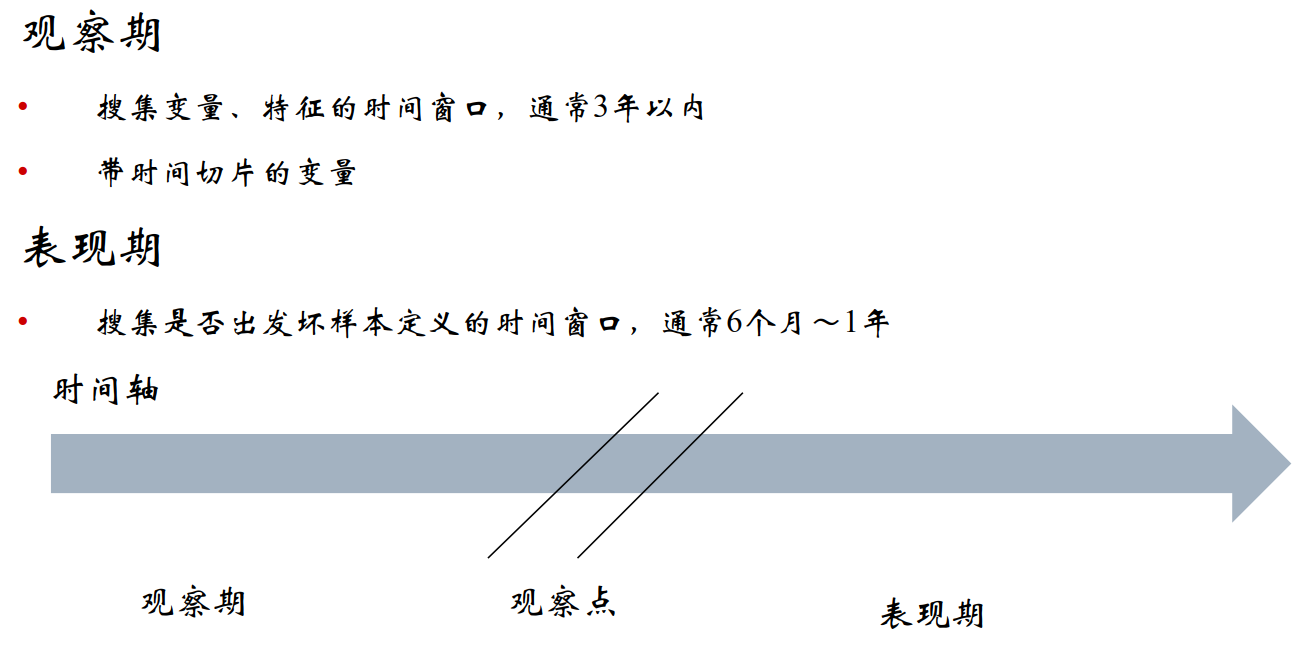观察点不一定是哪一天，可以是一段时间内，在某个时间区间内所有申请人，只要他们观察期和表现期相同即可。举例来说，当一个申请人在2017-7-14号这天来银行申请贷款，银行需要用已有的模型对申请人进行一个申请评分，评估他未来一年（表现期）内违约或者是逾期的概率，然后决定是否放贷。那么这个已有的模型是什么时候建立的呢？这里我们假定观察期为三年，因为上面是评估一年所以这里表现期为一年，那么往前推一年为2016-7-14号左右某个时间区间内作为观察点，再往前推三年（即观察期：2013-7-14到2016-7-14），利用这三年所有观察点内申请人一些信息建立模型的观察变量（即特征），然后再往后推一年（即表现期：2016-7-14到2017-7-14），所有在观察点内的申请人在这一年时间内的表现情况来定义违约。然后来训练出一个模型。对2017-7-14号的申请人进行评分。所以申请评分卡模型有着天然的滞后性，需要不断的对其模型进行监控。

3.评分卡模型的开发步骤
立项数据准备与预处理 银行自有数据和第三方数据模型构建 假设模型训练集的观察点（即客户的申请时间段）为2016-01到2016-03,那么这个模型的观察期（这里我们假定为三年）为2013-01到2016-01,模型的表现期（这里我们假定为一年）为2016-03到2017-03。模型评估 对照上面的模型构建的时间来，我们来建立测试集，假定其测试集观察点（即客户的申请时间段）为（2017-04),同理可得观察期，和表现期真实的违约或者逾期与否。这时把模型放在这个测试集上进行测试看看效果如何。这里需要注意训练集和测试集上用户在表现期的表现如何都是基于一个已经发生的时间段上。验证/审计模型部署 新旧模型替换，评分卡的实时性要求没那么高，在银行通常一个月更新一次模型。有些咨询机构可能一天更新一次评分卡模型。模型监控 跟踪模型各项性能是否发生弱化。
4.评分卡开发的常用模型
逻辑回归

优点：简单、稳定、可解释，技术成熟，易于检测与部署 缺点：准确度不高
决策树

优点：对数据的质量要求低，易解释 缺点：准确度不高
神经元模型组合模型（模型融合）

缺点：准确度高，不宜过拟合 缺点：不易解释；部署困难；计算量大

5.模型监控指标
AR(Accuracy Ratio) 该指标是衡量分数预测能力的指标，需要一个完整的表现期。取值位于-1~1之间。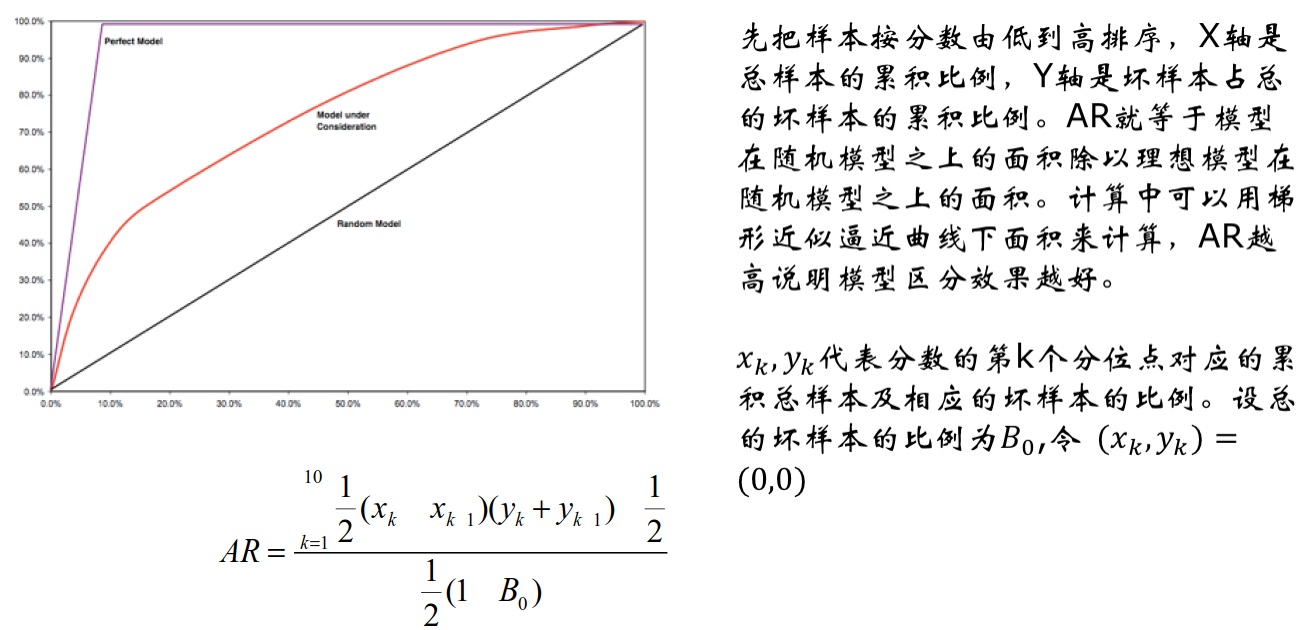KS(Kolmogorov-Smirnov) 该指标是衡量分数区分能力的指标。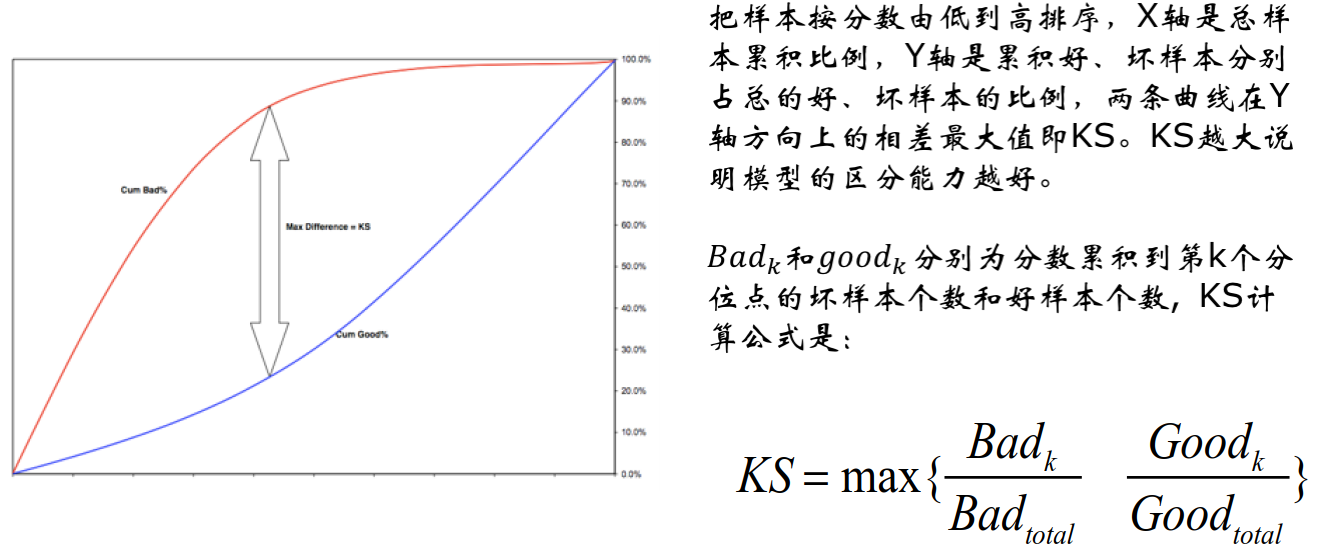PSI(Population Stability Index) 该指标是衡量分数稳定性的指标Kendalls Tau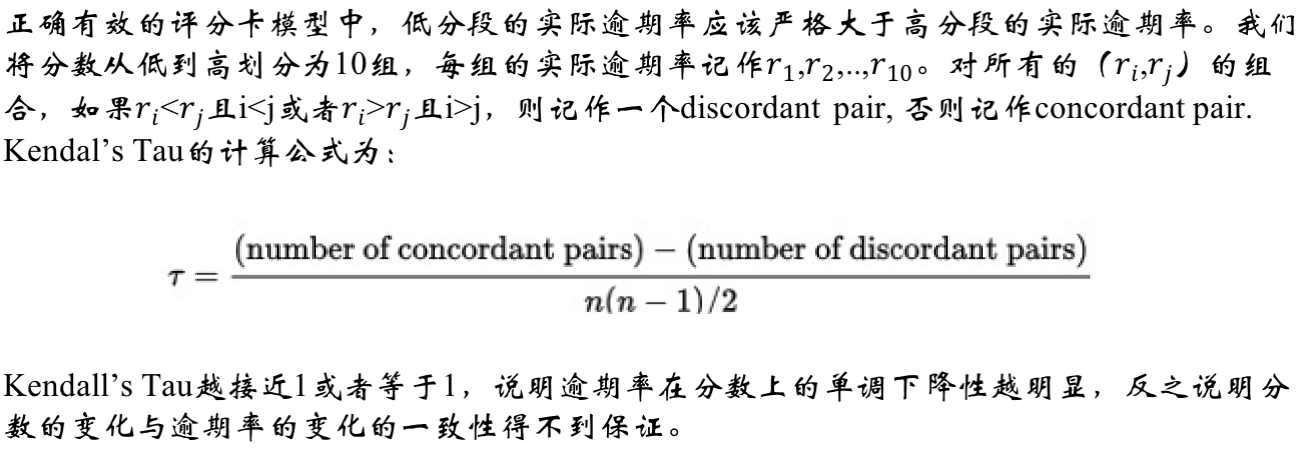Migration Matrix 迁移矩阵是衡量分数迁移的指标。二、申请评分卡在互联网金融业的重要性和特性
互联网金融机构可分为如下情形：
传统金融机构+非金融机构传统金融机构：传统金融业务的互联网创新以及电商化创新、APP软件等非金融机构 利用互联网技术进行金融运作的电商企业 （P2P）模式的网络借贷平台 众筹模型的网络投资平台 挖财类（模式）的手机理财APP 第三方支付平台
开发申请评分卡的作用：
风险控制营销资本管理
评分卡的特性：
稳定性区分性预测能力和逾期概率等价
三、贷款申请环节的数据介绍和描述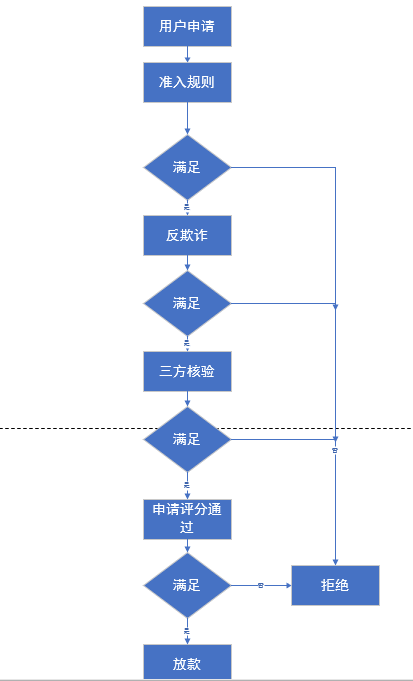1.申请评分卡的常用特征
个人信息 学历、性别、收入负债信息 在本金融机构或其他金融机构的负债情况消费能力 商品购买记录、出境游、奢侈品消费历史信用记录 历史逾期行为新兴数据 人际社交、网络足迹、出行、个人财务
2.数据介绍
每一行代表一个样本（一笔成功成交借款），每个样本包含200多个各类字段。 PPD_Training_Master_GBK_3_1_Training_Set：
idx：每一笔贷款的unique key  UserInfo_*：借款人特征字段  WeblogInfo_*:Info网络行为字段  Education_Info*:学历学籍字段  ThirdParty_Info_PeriodN_*:第三方数据时间段N字段  SocialNetwork_*:社交网络字段  LinstingInfo：借款成交时间  Target：违约标签(1 = 贷款违约，0=正常还款)
PPD_Userupdate_Info_3_1_Training_Set： 主要是借款人修改信息
ListingInfo1：借款成交时间UserupdateInfo1：修改内容UserupdateInfo2：修改时间
四、非平衡样本问题的定义和解决办法
在分类问题中，每种类别的出现概率未必均衡。
信用风险：正常客户远多于逾期/违约用户流失风险：留存客户多于流失客户
由于样本类别不均衡，训练出来的模型，会降低对少类样本的灵敏性，模型泛化能力差。
1.非平衡样本的解决办法
过采样

优点：简单，对数据质量要求不高 缺点：过拟合
欠采样

优点：简单，对数据质量要求不高 缺点：丢失重要信息
SMOTE(合成少数过采样技术)

优点：不易过拟合，保留信息 缺点：不能对有缺失值和类别变量做处理

SMOTE算法如下：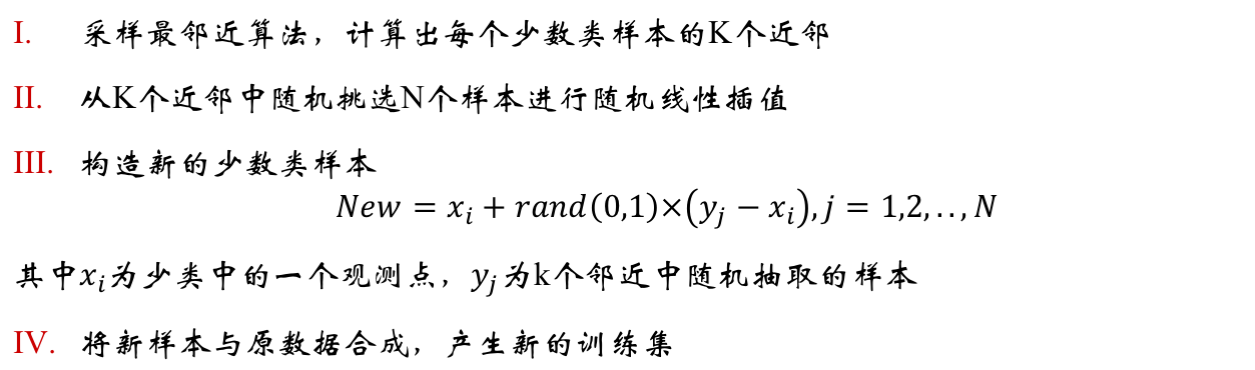展开全文• 对于选择逾期多少天以后的作为坏客户除了定性的与业务部门商定外，可以进行定量分析，建立滚动率二维分析表，观察起始点每个账龄在6个月后的迁徙情况，对于滚动有坏件率依然很高的账龄确定为坏用户，逾
1、确定建卡目标：区分申请的用户是好用户还是坏用户。
2、好坏用户定义：灵活设定，如逾期30天以上的为坏用户。这里还涉及表现期和观察期的问题，观察期是你选用哪个时段的样本入模，此处选择2018年全年作为观察期。表现期顾名思义就是用户借款后逾期表现多久趋于稳定，根据vintage分析，小微客户一般在放款后8~12月状态稳定。对于选择逾期多少天以后的作为坏客户除了定性的与业务部门商定外，可以进行定量分析，建立滚动率二维分析表，观察起始点每个账龄在6个月后的迁徙情况，对于滚动有坏件率依然很高的账龄确定为坏用户，逾期时间较短的账龄设为不确定客户。
3、根据业务需求，可以对用户分组、分别建立评分卡，为不同群体选择不同的变量能够增大模型的辨别力。
代码部分 1、引入需要的包
import numpy as np
import sys, os
import pandas as pd
from pandas import Series
from datetime import datetime, timedelta
import math
import matplotlib.pyplot as plt
import copy
from sklearn.feature_selection import RFE
from random import sample
from imblearn.over_sampling import SMOTE

2、读取数据
tongdun = pd.read_csv("D:/python/ysdthree/tongdun.csv", encoding="GBK")
...
yl = yl.loc[:7175,]

此处使用的主要是3方数据，大概有16个。加上平台放款的用户好坏标签。 使用pd.merge将读取的表合并成一个大宽表，有1219列。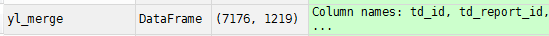3、做数据清理 去掉无关列、去掉全空列、去掉全为0或全为1的列，这些数据没有区分能力。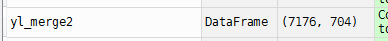剩下704个变量
有些变量的空值不是np.nan，而是空格或者-，需要做转化
for i in yl_merge2.columns:
yl_merge2[i] = yl_merge2[i].apply(lambda x:np.NaN if str(x).isspace() else x)
for i in yl_merge2.columns:
yl_merge2[i] = yl_merge2[i].apply(lambda x:np.NaN if str(x)=='-' else x)

#去掉空值大于60%的列
col_name = []
count = []
for i in yl_merge2.columns:
nan_counter = 0
for nan in yl_merge2[i].isnull():
if nan:
nan_counter +=1
col_name.append(i)
count.append(nan_counter)
count_list = pd.DataFrame({'col_name':col_name,'nan_count':count})
cut60 = len(yl_merge)*0.6
drop_60nan =  count_list[count_list['nan_count']>cut60].col_name

yl_merge3 = copy.deepcopy(yl_merge2)
yl_merge3 = yl_merge3.drop(drop_60nan,axis=1)

去掉后剩余227个变量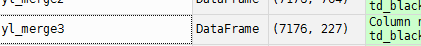此处的目标是做变量筛选，选出后续建模的变量，由于后续的计算消耗资源较大，前面先用简单的规则排除无用变量。
for i in col_time2:
yl_merge3[i] = pd.to_datetime(yl_merge3[i],format='%m/%d/%Y %H:%M:%S')
for i in col_time2:
yl_merge3[i] = yl_merge3['now'] - yl_merge3[i]
yl_merge3[i] = yl_merge3[i].apply(lambda x:x.days)

将时间转化为数值便于统一计算。
from sklearn.preprocessing import LabelEncoder
le = LabelEncoder()
yl_merge4['td_final_decision'] = le.fit_transform(yl_merge4['td_final_decision'])

对字符串变量进行编码
def calc_score_median(sample_set,var):
var_list = list(np.unique(sample_set[var]))
var_median_list = []
for i in range(len(var_list)-1):
var_median = (var_list[i] + var_list[i+1])/2
var_median_list.append(var_median)
return var_median_list

def choose_best_split(sample_set,var,min_sample):
score_median_list = calc_score_median(sample_set,var)
median_len = len(score_median_list)
sample_cnt = sample_set.shape
sample1_cnt = sum(sample_set['target'])
sample0_cnt =sample_cnt - sample1_cnt
Gini = 1 - np.square(sample1_cnt/sample_cnt)-np.square(sample0_cnt/sample_cnt)
bestGini = 0.0; bestSplit_point = 0.0; bestSplit_position = 0.0
for i in range(median_len):
left = sample_set[sample_set[var]<score_median_list[i]]
right = sample_set[sample_set[var]>score_median_list[i]]
left_cnt = left.shape; right_cnt = right.shape
left1_cnt = sum(left['target']); right1_cnt = sum(right['target'])
left0_cnt = left_cnt - left1_cnt; right0_cnt = right_cnt - right1_cnt;
left_ratio = left_cnt/sample_cnt; right_ratio = right_cnt/sample_cnt
if left_cnt < min_sample or right_cnt < min_sample:
continue

Gini_left = 1 - np.square(left1_cnt / left_cnt) - np.square(left0_cnt / left_cnt)
Gini_right = 1 - np.square(right1_cnt / right_cnt) - np.square(right0_cnt / right_cnt)
Gini_temp = Gini - (left_ratio * Gini_left + right_ratio * Gini_right)
if Gini_temp > bestGini:
bestGini = Gini_temp; bestSplit_point = score_median_list[i]
if median_len > 1:
bestSplit_position = i/(median_len - 1)
else:
bestSplit_position = i/median_len
else:
continue
Gini = Gini - bestGini
return bestSplit_point,bestSplit_position

def bining_data_split(sample_set,var,min_sample,split_list):
split,position = choose_best_split(sample_set,var,min_sample)
if split != 0.0:
split_list.append(split)
sample_set_left = sample_set[sample_set[var] < split]
sample_set_right = sample_set[sample_set[var] > split]
if len(sample_set_left) >= min_sample * 2 and position not in [0.0,1.0]:
bining_data_split(sample_set_left,var,min_sample,split_list)
else:
None
if len(sample_set_right) >= min_sample * 2 and position not in [0.0,1.0]:
bining_data_split(sample_set_right,var,min_sample,split_list)
else:
None

def get_bestsplit_list(sample_set,var):
min_df = sample_set.shape * 0.1
split_list = []
bining_data_split(sample_set,var,min_df,split_list)
split_list.append(sample_set[var].min()-0.5)
split_list.append(sample_set[var].max())
return split_list

使用CART决策树进行最优分箱,算法分几个模块：1、计算变量值中每两个值的中间值作为备选切分点。2、选择最优切分点，遍历1中的备选切分点并计算其gini系数=1-1占比的评分-0占比的平方。切分后的gini系数最小的点为选出来的最优切分点。3、对切分后的左右两边反复调用2，直到达到停止条件：达到最小bin如大于样本量的0.2，最多便能获得5组。4、调用3获得所有的切分点，在切分点的集合中加入变量的最大值和最小值。 调用此函数可得各变量的分箱点，使用pd.cut分别对各变量进行切分。 分箱只针对于连续性变量且数值较多的变量如多于10个。
对变量进行woe转化 对于非数值的变量如分类变量和刚分箱产生的区间变量。woe=log(坏的占比/好的占比），woe转换的作用有统一量纲、去掉非线性等，相当于数据标准化。
def get_woe_data(cut):
woel=[]
valuel=[]
for i in yl_merge5[cut].unique():
good_distr = (good_num + 1e-5)/(total_good + 1e-5)
woel.append(woe)
valuel.append(i)
Woe = pd.DataFrame({'value':valuel,'woe':woel})
Woe = Woe.sort_values('value',ascending=True)
Woe = Woe.reset_index(drop=True)
return Woe

继续筛选变量 计算各变量间相关性，用pandas的.corr函数计算 r=协方差/各自标准差之积
计算IV值=sum(好的占比-坏的占比）*woe，一般IV值大于0.04是可用的，大于0.3说明预测能力强，0.1~0.3有部分预测能力。
此处保留IV至大于0.04的变量，剩下96个。
下一步进行建模：使用逻辑回归模型、使用sklearn的递归特征选择来进行变量选择，算法是根据选择的estimator计算每个变量的重要性如coef_，feature_importances_，每次去掉最不重要的变量后继续训练继续去掉最不重要的变量，直到到达规定的变量数。n_features_to_select=16最终选择16个变量进行建模。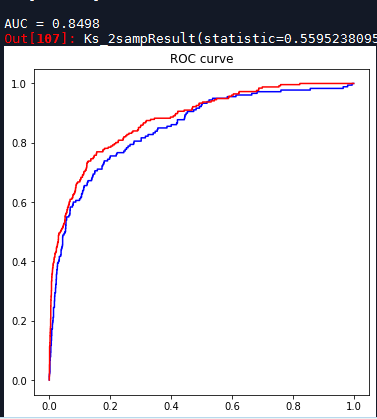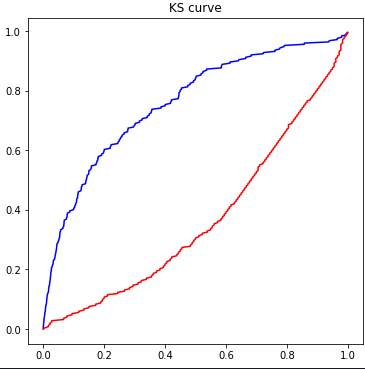结果得到AUC=0.84，是ROC曲线下的面积，横轴是漏杀率，纵轴是误杀率。好的AUC反应了能在低漏杀率（拒绝掉大部分坏客户）的情况下保持高误杀率（让好大部分客户通过），说明模型结果对好坏样本的区分度大。AUC在0.7-0.8代表可以接受的区分度，0.8-0.9代表非常好的区分度，0.9以上有过拟合的危险。 ks=0.56，横轴是违约概率，纵轴是累计客户比例。ks值是累计好客户比例和坏客户比例的最大差值。ks在0.3~0.5代表可以接受的区分度，0.5-0.75代表非常好的区分度。大于0.75代表有过拟合的危险。
factor = 20 / np.log(2)
offset = 600 - 20 * np.log(20) / np.log(2)
coe =  rfe.estimator_.coef_
intercept = rfe.estimator_.intercept_

def get_score(coe,woe,factor):
scores=[]
for w in woe:
score=round(coe*w*factor,0)
scores.append(score)
return scores

def compute_score(series,bins,score):
list = []
i = 0
while i < len(series):
value = series[i]
j = len(bins) - 2
m = len(bins) - 2
while j >= 0:
if value >= bins[j]:
j = -1
else:
j -= 1
m -= 1
list.append(score[m])
i += 1
return list

最后建立评分卡 score = A + B * ln(Odds),B=PDO/ln(2)，如设PDO=20则表示每增加20分，Odds加倍。设平均分为600，计算出A即为截距，基础分。Odds= -woe该变量的系数B。
得到分数后便可使用分数来做客户分级或选择决策点拒绝客户。
展开全文机器学习 数据分析
• 信贷评分卡模型基础知识一、模型输出概率转化为分数 一、模型输出概率转化为分数 风控模型（XGB模型、LGB模型或LR模型）直接输出的是客户逾期概率，在评分卡应用场景中，需要将此概率转化为对客户的评分。转化方式...


信贷评分卡模型基础知识
一、模型输出概率转化为分数二、模型KS指标

一、模型输出概率转化为分数

o

d

d

s

=

p

1

−

p

odds = \frac{p}{1-p}

评分卡分数可表示为下式：

s

c

o

r

e

=

A

−

B

∗

l

o

g

(

o

d

d

s

)

=

A

−

B

∗

l

o

g

(

p

1

−

p

)

\begin{aligned} score &=A-B*log(odds)\\ &=A-B*log(\frac{p}{1-p}) \end{aligned}

其中，A和B是常数。式中的负号使得违约概率越低，得分越高。通常情况下，这是分值的理想变动方向，即高分值代表低风险，低分值代表高风险。
常数A、B的值可以通过将两个已知或假设的分值带入计算得到。通常情况下，需要设定两个假设： （1）给定odds=Ratio时，预期分数为Base； （2）Ratio翻倍时，分数减少值为PDO（即Point of Double Odds）
于是，可以得到二元一次方程组

{

B

a

s

e

=

A

−

B

∗

l

o

g

(

R

a

t

i

o

)

B

a

s

e

−

P

D

O

=

A

−

B

∗

l

o

g

(

2

∗

R

a

t

i

o

)

\begin{cases} Base&= A-B*log(Ratio)\\ Base- PDO&=A-B*log(2*Ratio) \end{cases}

由此可求解得到A和B的值

B

=

P

D

O

/

l

o

g

(

2

)

A

=

B

a

s

e

+

B

∗

l

o

g

(

R

a

t

i

o

)

\begin{aligned} B &= PDO / log(2)\\ A&=Base + B * log(Ratio) \end{aligned}

举例 我们设定

o

d

d

s

=

1

/

15

odds=1/15

，即

p

b

a

d

/

p

g

o

o

d

=

1

/

15

时，评分为600。当

o

d

d

s

odds

每增大一倍，评分减60分，即PDO=60。由此我们可以得到

B

=

60

/

l

o

g

(

2

)

≈

86.56

B=60/log(2)\approx86.56

，

A

=

600

+

60

l

o

g

(

1

/

15

)

/

l

o

g

(

2

)

≈

365.59

A=600+60log(1/15)/log(2)\approx365.59

。于是可以得到概率p和评分score的转换公式为

s

c

o

r

e

=

365.59

−

86.56

∗

l

o

g

(

p

/

(

1

−

p

)

)

score=365.59-86.56*log(p/(1-p))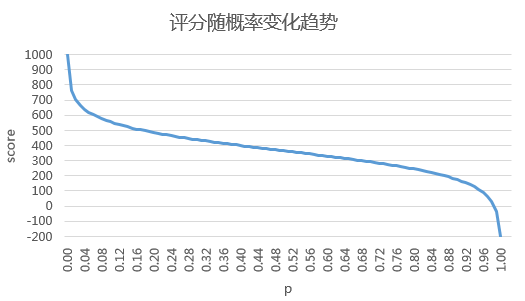python代码
import numpy as np

def p_to_score(p, PDO=60.0, Base=600, Ratio=1.0/15.0):
"""
逾期概率转换分数
:param p: 逾期概率
:param PDO: points double odds. default = 60
:param Base: base points. default = 600
:param Ratio: odds. default = 1.0/15.0
:returns: 模型分数
"""
B = PDO / np.log(2)
A = Base + B * np.log(Ratio)
score = A - B * np.log(p / (1 - p))
return round(score, 0)

二、模型KS指标

K

S

=

m

a

x

∣

P

c

u

m

(

B

a

d

)

−

P

c

u

m

(

G

o

o

d

)

∣

KS是最主要的模型评价指标， KS越高，模型越好。但过高的KS可能意味着过度拟合从而导致模型不稳定。通常能达到40%以上的模型就很不错了。数据较差时，20%的KS也勉强可以用。
举例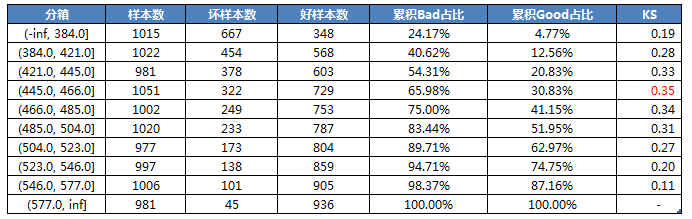对应的KS曲线如下图所示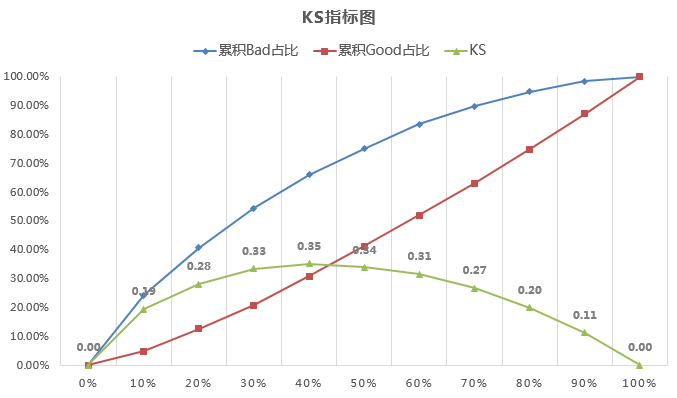`
展开全文• 本篇我们来学习一下行为评分卡(B卡)，首先什么是行为评分卡呢，行为评分卡的使用场景以及目的，适用的信贷产品？ 其中特别注意一下，不适合先息后本的信贷产品，因为每个月的违约概率不一样，不好预测 观察...观察期表现期
• # 固定资产贷款量大于50的是超级离群数据 # 处理异常值：过滤离群值，筛选出剩余部分数据 df1 = df1 [ df1 [ '可用额度比值' ] < 1 ] df1 = df1 [ df1 [ '年龄' ] > 0 ] df1 = df1 [ df1 [ '逾期30-59天...python 逻辑回归 二分类
• 1、定义：B卡即行为评分（Behavior Scoring），主要目的为监控放款后的风险变化。 2、业务阶段：B卡适用于贷...3、数据使用：B卡行为评分卡则是在申请人有了贷款还款行为后，有相当的数据基础后进行的分析，一般为3-...
• （十）催收评分卡的介绍 评分卡可分为申请评分卡(A卡)、行为评分卡(B卡)、催收评分卡(C卡)。不同的卡使用场景不一样...要学习催收评分卡，首先要先了解一下信贷客户管理的周期 其实对于逾期，不能一棍子打死，逾...还款率模型
• 风控业务背景 不夸张地说，贷前风控可覆盖80%的风险，这是业内普遍共识，当然更是信贷风控的内在需要，毕竟放款...本文以贷前授信风控模型为例，介绍客户层申请评分卡的一些构建思路。 目录 Part 1. 产品层和客户层评分大数据 数据分析
• 本文主要讲“变量选择”“模型开发”“评分卡创建和刻度” 变量分析 首先，需要确定变量之间是否存在共线性，若存在高度相关性，只需保存最稳定、预测能力最高的那个。需要通过 VIF(variance inflation ...Logistic
• 评分卡模型1 消费信贷概述1.1 消费金融概述1.2 消费信贷发展痛点1.3 痛点解决思路2 模型业务目标确定2.1 风控内容2.2 Vintage分析法2.3 如何确定业务目标2.4 Vintage和迁移率模型对比3 A卡和B卡3.1 A卡3.2 B卡3.3 C...
• 本帖是在2019年5月初入门python之时，选取的较为系统的练手案例，主要内容是信用风险计量体系之主体评级模型的开发过程（可用“四张卡”来表示，分别是A、B、C和F）。 如今再回顾，结合前几月股票市场被割...Python
• 我们的评分卡上线后，如何对评分卡的效果进行有效监测，监测哪些指标，监测的指标阈值达到多少我们需要对现有评分卡进行调整更新？这是我们在评分卡上线后需要持续性监测、关注的问题，今天就来跟大家分享一下互金...PSI 模型稳定性
• 从今天开始本号会陆续公开催收评分卡的原创教程和资料，如果对这部分内容有兴趣，麻烦动动手关注转发，谢谢大家的捧场。 搜索关注公众号“ 番茄风控大数据”，获取更多风控干货。   我这里所要介绍给各位的是：...python
• 如今在银行、消费金融公司等各种贷款业务机构，普遍使用信用评分，对客户实行打分制，以期对客户有一个优质与否的评判。但是不是所有人都知道信用评分卡还分A,B,C卡三类！A卡（Application score card）申请评分卡B...
• 风险评分、收入评分、响应度评分客户流失（忠诚度）评分、催收评分、信用发卡审核评分、房屋按揭贷款发放审核评分、信用额度核定评分等。 综合信用风险评分——鹏元 800 2005年4月底，鹏元征信有限公司自主研发...
• 什么是评分卡信贷场景中） 以分数的形式来衡量风险几率的一种手段 对未来一段时间内违约/逾期/失联概率的预测 通常评分越高越安全 根据使用场景分为反欺诈评分卡、申请评分卡、行为评分卡、催收评分卡 为什么...KS ROC
• 一、评分卡介绍 二、数据收集 三、数据准备 3.1. 数据准备包括：处理异常值、缺失值、定义好坏客户、样本量、定义样本窗口期等。 3.2. 变量可视化：可助于寻找特征数据之间的关系和发现问题。 四、变量筛选 ...
• （六）评分卡知识查漏补缺 制作评分卡时候，做变量相关性应该在哪一个步骤？ 在变量WOE编码之后，因为变量可能在WOE编码前存在相关性，不过编码过后就不存在相关性了；同理有一些变量在WOE编码前不相关性，但是...
• （七）申请评分卡模型Python实现（图文+代码实现） 贷前准入环节流程图大致如下 为什么需要建立评分卡？ 所有的模型一定是服务于业务的，那么业务上到底出现了什么问题，需要用到评分卡模型去解决呢？我们先从...
• 某网贷行业贷款，包括信用违约标签（因变量）、建模所需的基础与加工字段（自变量）、相关用户的网络行为原始数据，关于信用评分模型的应用以及实现，进行信用评分卡构建，以此来对客户贷款进行风险预警。
• 文章目录申请评分卡行为评分卡催收评分卡反欺诈模型 ...除此之外还有反欺诈模型、营销评分卡客户流失评分卡等，它们在风控系统中的先后顺序如图 1-2 所示 申请评分卡 申请评分卡是平台风险管理中最
• 在银行借贷场景中，评分卡是一种以分数形式来衡量一个客户的信用风险大小的手段，它衡量向别人借钱的人（受信人，需要融资的公司）不能如期履行合同中的还本付息责任，并让借钱给别人的人（授信人，银行等金融机构...数据分析
• ## C卡催收评分卡

千次阅读 2020-03-26 21:22:27
转自公众号：天天学风控 1、定义：C卡（Collection scorecard）催收评分...3、数据使用：A卡申请评分卡一般可做贷款0-1年的信用分析，B卡行为评分卡一般为3-5年，催收评分卡则对数据要求更大，需加入催收后客户反应......